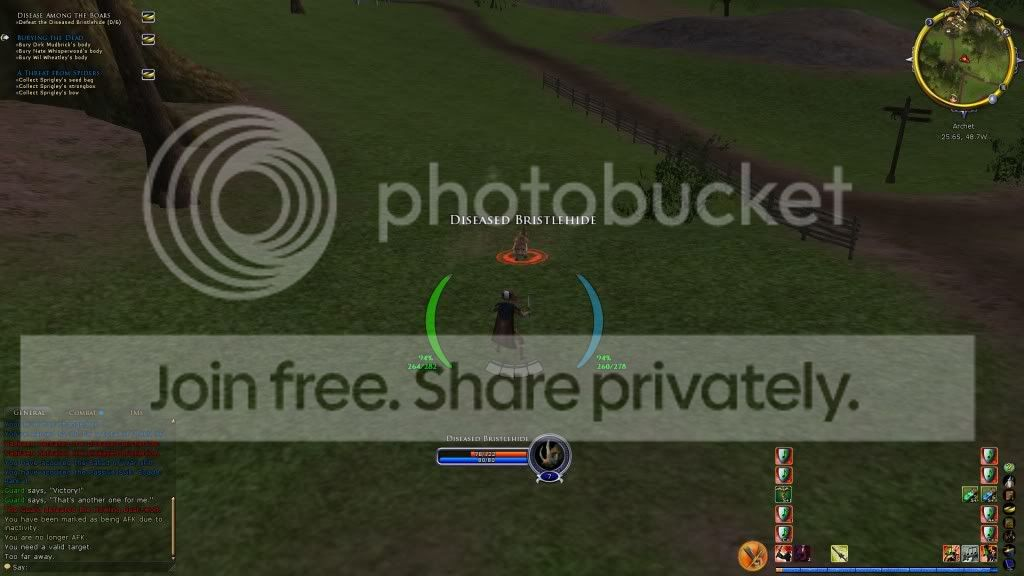LoTROInterface (https://www.lotrointerface.com/forums/index.php)
-   Pre-Beta Interfaces (L) (https://www.lotrointerface.com/forums/forumdisplay.php?f=17)
-   -   Domedian Minimalist UI (https://www.lotrointerface.com/forums/showthread.php?t=1076)

 Domedian 09-23-2010 08:42 PM

Domedian Minimalist UI

So I began asking for a UI Request:

But I just hate to ask someone to do the work for me, knowing that I could eventually figure things out...

I've been able to figure things out using a bit of code from Ray UI 2.2, thanks again Ray!

Problem:The only problem now, is I can;t figure out how to get the xp bar to work correctly. It is still tracking xp, but using the normal size increments, in a smaller bar.

I believe this is the needed bit of Code:

Code:

```<PanelFile ID="toolbar">                     <Element ID="ToolbarField"                                        X="0"    Y="&SCREENHEIGHT;" Width="&SCREENWIDTH;" Height="211" Detach="1">                             <Element ID="ToolbarFieldMain"                                        X="1450"    Y="11"  Width="420" Height="200" />                                  <Element ID="LevelMeter"                                        X="1450"  Y="187" Width="420" Height="20">                                                                        <Element ID="LevelMeter_LevelUpXP_TutorialHighlight"    X="0"    Y="0"  Width="420" Height="20" />                 <Element ID="LevelMeter_Experience_TutorialHighlight"  X="0"    Y="0"  Width="420" Height="20" />                                      <Element ID="LevelMeterText"                                X="0"    Y="0"  Width="420" Height="20" />                 <Element ID="LevelMeterFill"                                X="0"    Y="0"  Width="420" Height="20" />                       <Element ID="LevelMeter_BonusMeter"                        X="0"    Y="0"  Width="420" Height="20" />                 <Element ID="LevelMeterBg"                                X="0"    Y="0"  Width="420" Height="20" />                 <Element ID="LevelMeter_Overlay"                        X="0"    Y="0"  Width="420" Height="20" />                               </Element>```
Current Screenshot:Any suggestions or feedback would be greatly appreciated,
Dom

Edit: 1. code and thanks
2. screenshot

 Brode 10-15-2010 02:07 PM

did anyone ever help you out with this? id sure like a bottom bar setup just like that.

 All times are GMT -5. The time now is 12:23 AM.